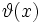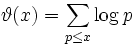# First Chebyshev function

View other such summations

## Definition

Letbe a positive real number. The first Chebyshev function of, denotedor, is defined as:,

where the sum is only over the prime numbers less than or equal to.

## Relation with other counting functions

• Prime-counting function: Denoted, this simply counts the number of primes less than or equal to.
• Second Chebyshev function: A similar summation, but this time of the von Mangoldt function over all prime powers less than or equal to.